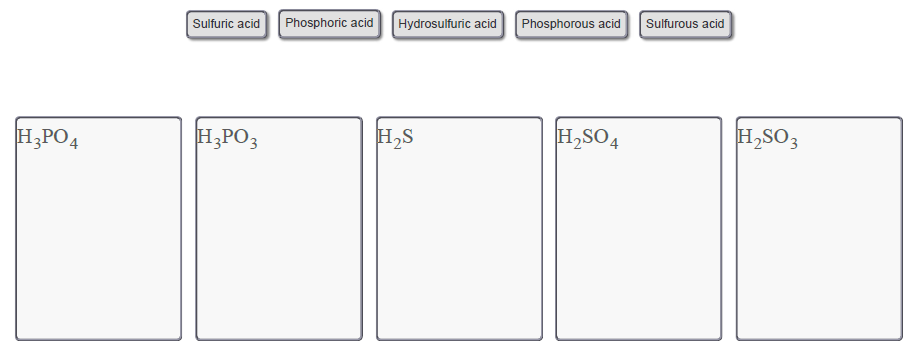Problem: In the Larger Molecules page of the simulation, when a molecule is created the name will display. Some acids can be created, demonstrating how this naming system works.Match each of the chemical formulas to the correct name of the acid.Drag the appropriate items to their respective bins.Acid nomenclatureThe name of an acid can be derived from the name of the anion that is bonded to one or more atoms of H + to neutralize the charge.Anions are named according to the following rules:• For monatomic anions, the ending is replaced with "ide." For example, F − is named fluoride and O2 − is named oxide.• Some polyatomic anions contain oxygen. If an element can only form two oxyanions then the one with the least amount of oxygens is given the ending "ite." The one containing the most oxygens is given the ending "ate."• Elements that can form four polyatomic oxyanions use prefixes in addition to the "ite" and "ate" endings. The oxyanion with the least amount of oxygens is given the prefix "hypo" and the ending "ite" (i.e., ClO− is hypochlorite). The oxyanion with the second least amount of oxygens is simply given the "ite" ending (i.e., ClO2− is chlorite). The oxyanion with the second most amount of oxygens is simply given the "ate" ending (i.e., ClO3− is chlorate). Finally, the oxyanion with the most amount of oxygens is given the prefix "per" and the ending "ate" (i.e., ClO4− is perchlorate).When naming the acid of the anion the prefix "hydro" is added to anions ending with "ide" and the endings of the anion are modified as follows:• "ide" is changed to "ic," and the prefix "hydro" is added.• "ate" is changed to "ic" (retain prefixes).• "ite" is changed to "ous" (retain prefixes).The word acid is added following the new name of the anion to complete the name of the acid.

FREE Expert Solution
82% (266 ratings)Problem Details

In the Larger Molecules page of the simulation, when a molecule is created the name will display. Some acids can be created, demonstrating how this naming system works.

Match each of the chemical formulas to the correct name of the acid.

Drag the appropriate items to their respective bins.Acid nomenclature

The name of an acid can be derived from the name of the anion that is bonded to one or more atoms of H + to neutralize the charge.
Anions are named according to the following rules:

• For monatomic anions, the ending is replaced with "ide." For example, F − is named fluoride and O2 − is named oxide.
• Some polyatomic anions contain oxygen. If an element can only form two oxyanions then the one with the least amount of oxygens is given the ending "ite." The one containing the most oxygens is given the ending "ate."
• Elements that can form four polyatomic oxyanions use prefixes in addition to the "ite" and "ate" endings. The oxyanion with the least amount of oxygens is given the prefix "hypo" and the ending "ite" (i.e., ClO is hypochlorite). The oxyanion with the second least amount of oxygens is simply given the "ite" ending (i.e., ClO2 is chlorite). The oxyanion with the second most amount of oxygens is simply given the "ate" ending (i.e., ClO3 is chlorate). Finally, the oxyanion with the most amount of oxygens is given the prefix "per" and the ending "ate" (i.e., ClO4 is perchlorate).

When naming the acid of the anion the prefix "hydro" is added to anions ending with "ide" and the endings of the anion are modified as follows:

• "ide" is changed to "ic," and the prefix "hydro" is added.
• "ate" is changed to "ic" (retain prefixes).
• "ite" is changed to "ous" (retain prefixes).

The word acid is added following the new name of the anion to complete the name of the acid.